## Identifying Trends of a Graph

### Learning outcome

• Recognize the trend of a graph

Data from the real world typically does not follow a perfect line or precise pattern. However, depending on the data, it does often follow a trend. Trends can be observed overall or for a specific segment of the graph. When looking a graph to determine its trend, there are usually four options to describe what you are seeing.

### Graph Trends

• One variable increases as the other increases
• One variable decreases as the other increases
• There is no change in one variable as the other increases or decreases
• The data is so scattered and random that no trend can be determined from the graph

Let’s take a look at some graph depicting real data and see what we can determine about their trends.

### Example

The graph below shows the closing value in (\$) of the Dow Jones index in relation to the year. What is the overal trend of this data?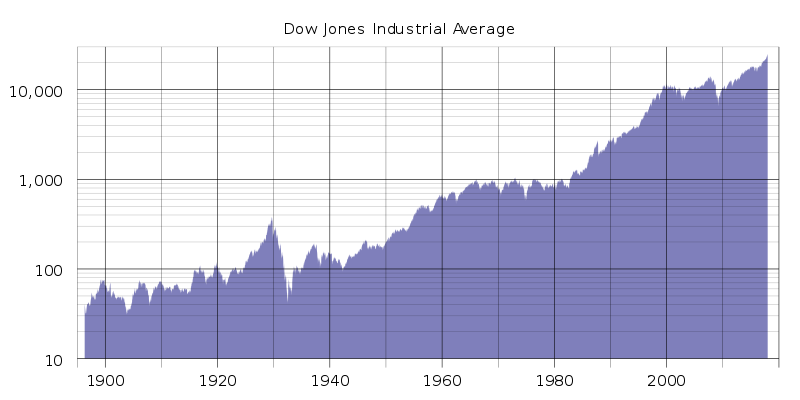Though the points don’t create a perfect line, if you hold your pencil over the data points, you can see that a diagonal line going up to the right is formed. If we pick pick a point from each end we can analyze the values. In $1920$ the Dow Jones was at about $100$. In $2000$ the Dow Jones was at about $10,000$. So as the years increased by $80$, the value of the index increased by $9,900$.

We can say that the data on this graph fits the trend — “one variable increases as the other increases”.

As time passes, or the years increase, the value of the Dow Jones also increases.

### TrY IT

Now let’s look at a graph that show the global temperature differences collected over the 100+ years. How would you describe the trend of this graph?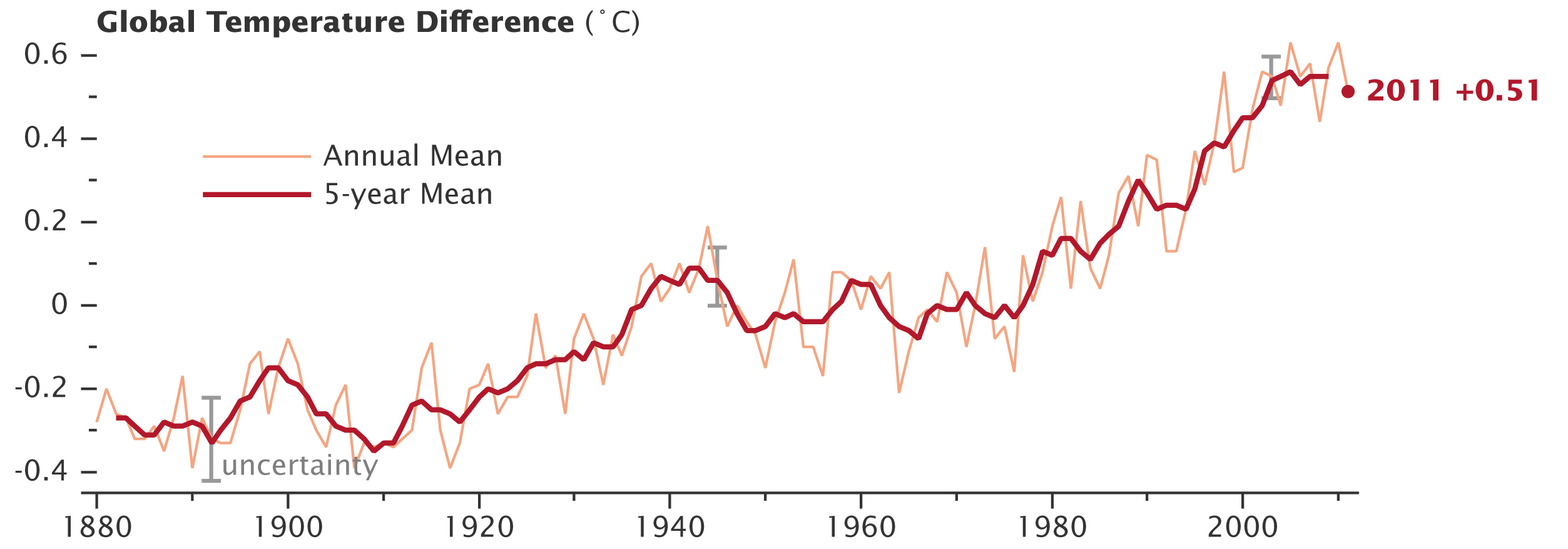### ExAMPLE

The following graph shows the fertility rate in various regions in a hundred year range.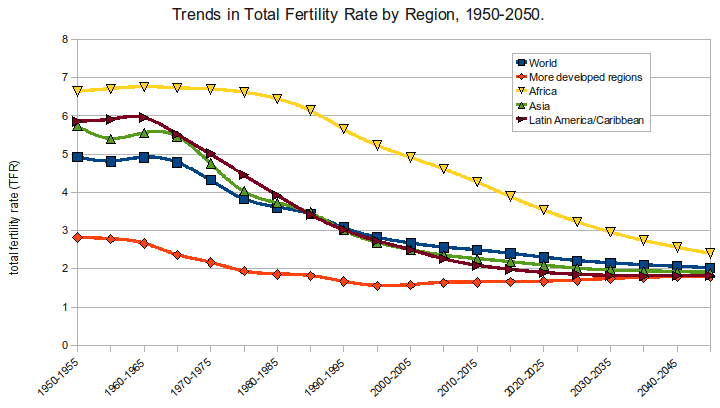Looking at this graph we can observe overall trends, individual regions, or segments of time.

1. What would you say is the overall trend of this data (Worldwide)?
2. What is the trend in more developed regions (red line w/ diamond points)?

### TRY IT

The graph below show the marriage rates in Great Britain over the past $80$ years.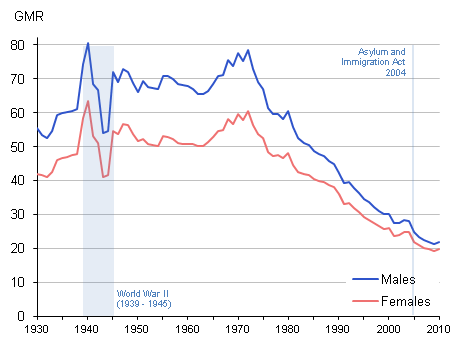Looking at this graph answer the following questions.

1. What is the trend of this data for males between $1940$ and $1975$?
2. What is the trend of this data for females between $1980$ and $2010$?

### Example

Look at the data points scattered all over the graph below. It’s possible that if a statistician analyzed the numbers, there is a slight trend. However, based on our knowledge and the data provided, we cannot tell how median household income is related to overrepresentation.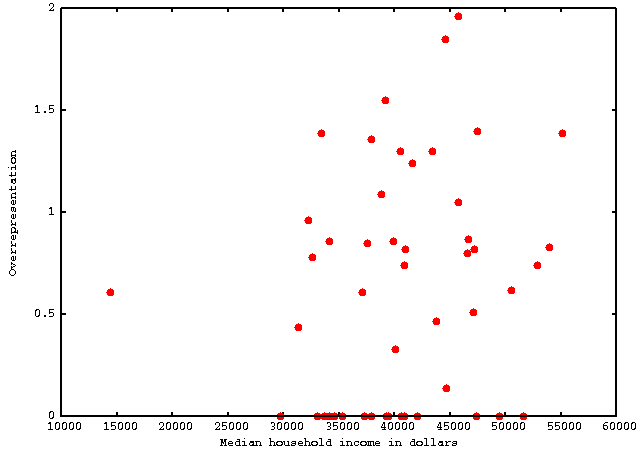We would say that we cannot determine the trend of this data based on the graph.

## Contribute!

Did you have an idea for improving this content? We’d love your input.